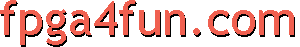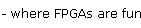Counters 2 - Special counters

Modulus counters

A modulus counter is a binary counter that rolls back before its natural end value. For example, let's say you want a modulus 10 counter (counts from 0 to 9), you can write this.

reg [3:0] cnt = 0;  // we need 4 bits to be able to reach 9

always @(posedge clk)
if(cnt==9)
cnt <= 0;
else
cnt <= cnt+1;
or this (a little more compact)
reg [3:0] cnt = 0;
always @(posedge clk) cnt <= (cnt==9) ? 0 : cnt+1;

Now a little of (free) optimization is available if you realize that we don't actually need to compare all the 4 bits of the counter to 9. The code below uses only bit 0 and bit 3 in the comparison.

always @(posedge clk) cnt <= ((cnt & 9)==9) ? 0 : cnt+1;
Gray counters

A Gray counter is a binary counter where only one bit changes at a time.
Here's how a 4bit Gray counter goes.

0000
0001
0011
0010
0110
0111
0101
0100
1100
1101
1111
1110
1010
1011
1001
1000
and then wraps back to 0000...

Gray code is useful to send values across clock domains (this way it has an uncertainty of only 1).

The easiest way to create a Gray counter is to first make a binary counter, and then convert the value to Gray.

module GrayCounter(
input clk,
output [3:0] cnt_gray
);

reg [3:0] cnt = 0;
always @(posedge clk) cnt <= cnt+1;  // 4bit binary counter

assign cnt_gray = cnt ^ cnt[3:1];  // then convert to gray
endmodule

It is also possible to create a native Gray counter.

module GrayCounter(
input clk,
output reg [3:0] cnt_gray = 0
);

wire [3:0] cnt_cc = {cnt_cc[2:1] & ~cnt_gray[1:0], ^cnt_gray, 1'b1};  // carry-chain type logic
always @(posedge clk) cnt_gray <= cnt_gray ^ cnt_cc ^ cnt_cc[3:1];
endmodule